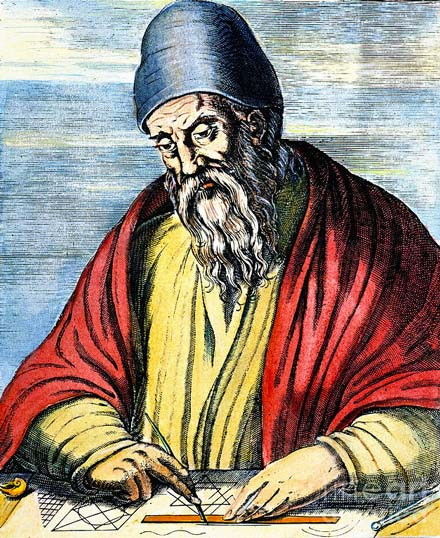## Glossaire

Sélectionnez l'un des mots clés à gauche…

# Euclidean GeometryEuclid’s Axioms

Temps de lecture: ~25 min

Before we can write any proofs, we need some common terminology that will make it easier to talk about geometric objects. These are not particularly exciting, but you should already know most of them:

A point is a specific location in space. Points describe a position, but have no size or shape themselves. They are labelled using capital letters.

In Mathigon, large, solid dots indicate interactive points you can move around, while smaller, outlined dots indicate fixed points which you can’t move.

A line is a set of infinitely many points that extend forever in both directions. Lines are always straight and, just like points, they don’t take up any space – they have no width.

Lines are labeled using lower-case letters like a or b. We can also refer to them using two points that lie on the line, for example PQ or QP. The order of the points does not matter.

A line segment is the part of a line between two points, without extending to infinity. We can label them just like lines, but without arrows on the bar above: AB or BA. Like, before the order of the points does not matter.

A ray is something in between a line and a line segment: it only extends to infinity on one side. You can think of it like sunrays: they start at a point (the sun) and then keep going forever.

When labelling rays, the arrow shows the direction where it extends to infinity, for example AB. This time, the order of the points does matter.

A circle is the collection of points that all have the same distance from a point in the center. This distance is called the radius.

## Congruence

These two shapes basically look identical. They have the same size and shape, and we could turn and slide one of them to exactly match up with the other. In geometry, we say that the two shapes are congruent.

The symbol for congruence is , so we would say that AB.

Here are a few different geometric objects – connect all pairs that are congruent to each other. Remember that more than two shapes might be congruent, and some shapes might not be congruent to any others:

Two line segments are congruent if they . Two angles are congruent if they (in degrees).

Note the that “congruent” does not mean “equal”. For example, congruent lines and angles don’t have to point in the same direction. Still, congruence has many of the same properties of equality:

• Congruence is symmetric: if XY then also YX.
• Congruence is reflexive: any shape is congruent to itself. For example, AA.
• Congruence is transitive: if XY and YZ then also XZ.

## Parallel and Perpendicular

Two straight lines that never intersect are called parallel. They point into the same direction, and the distance between them is always .

A good example of parallel lines in real life are railroad tracks. But note that more than two lines can be parallel to each other!

In diagrams, we denote parallel lines by adding one or more small arrows. In this example, abc and de. The symbol simply means “is parallel to”.

The opposite of parallel is two lines meeting at a 90° angle (right angle). These lines are called perpendicular.

In this example, we would write a b. The symbol simply means “is perpendicular to”.

## Euclid’s Axioms

Greek mathematicians realised that to write formal proofs, you need some sort of starting point: simple, intuitive statements, that everyone agrees are true. These are called axioms (or postulates).

A key part of mathematics is combining different axioms to prove more complex results, using the rules of logic.

The Greek mathematician Euclid of Alexandria, who is often called the father of geometry, published the five axioms of geometry:Euclid of Alexandria

First Axiom
You can join any two points using exactly one straight line segment.

Second Axiom
You can extend any line segment to an infinitely long line.

Third Axiom
Given a point P and a distance r, you can draw a circle with centre P and radius r.

Fourth Axiom
Any two right angles are congruent.

Fifth Axiom
Given a line L and a point P not on L, there is exactly one line through P that is parallel to L.

Each of these axioms looks pretty obvious and self-evident, but together they form the foundation of geometry, and can be used to deduce almost everything else. According to none less than Isaac Newton, “it’s the glory of geometry that from so few principles it can accomplish so much”.

Euclid published the five axioms in a book “Elements”. It is the first example in history of a systematic approach to mathematics, and was used as mathematics textbook for thousands of years.

One of the people who studied Euclid’s work was the American President Thomas Jefferson. When writing the Declaration of Independence in 1776, he wanted to follow a similar approach. He begins by stating a few, simple “axioms” and then “proves” more complex results:

“We hold these truths to be self-evident: that all men are created equal, that they are endowed by their Creator with certain unalienable Rights, that among these are Life, Liberty and the pursuit of Happiness.”

We, therefore … declare, that these United Colonies are, and of right ought to be, free and independent states.”

This is just one example where Euclid’s ideas in mathematics have inspired completely different subjects.

Archie